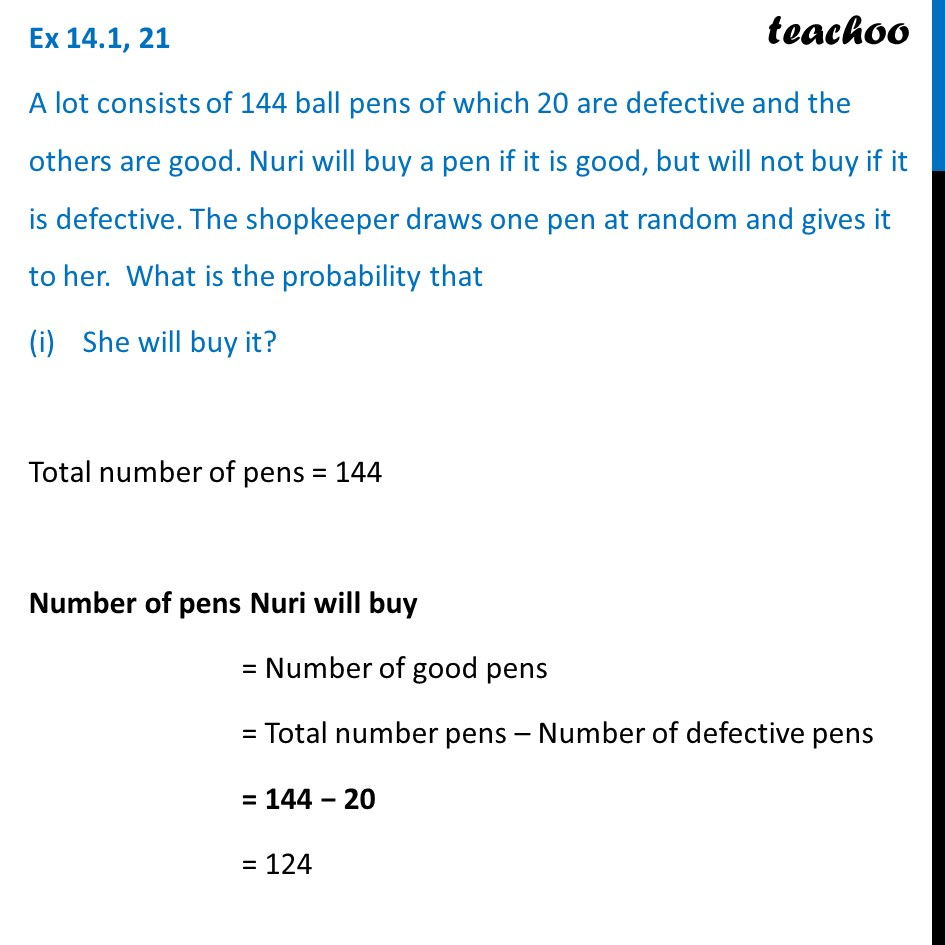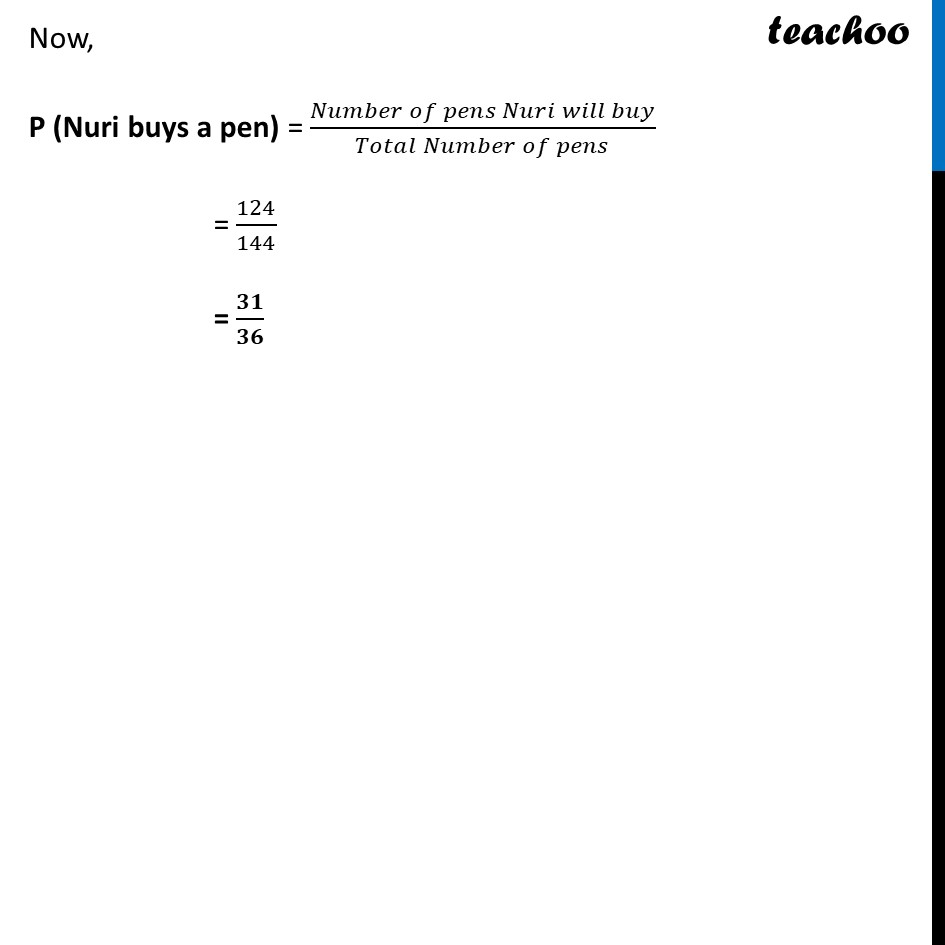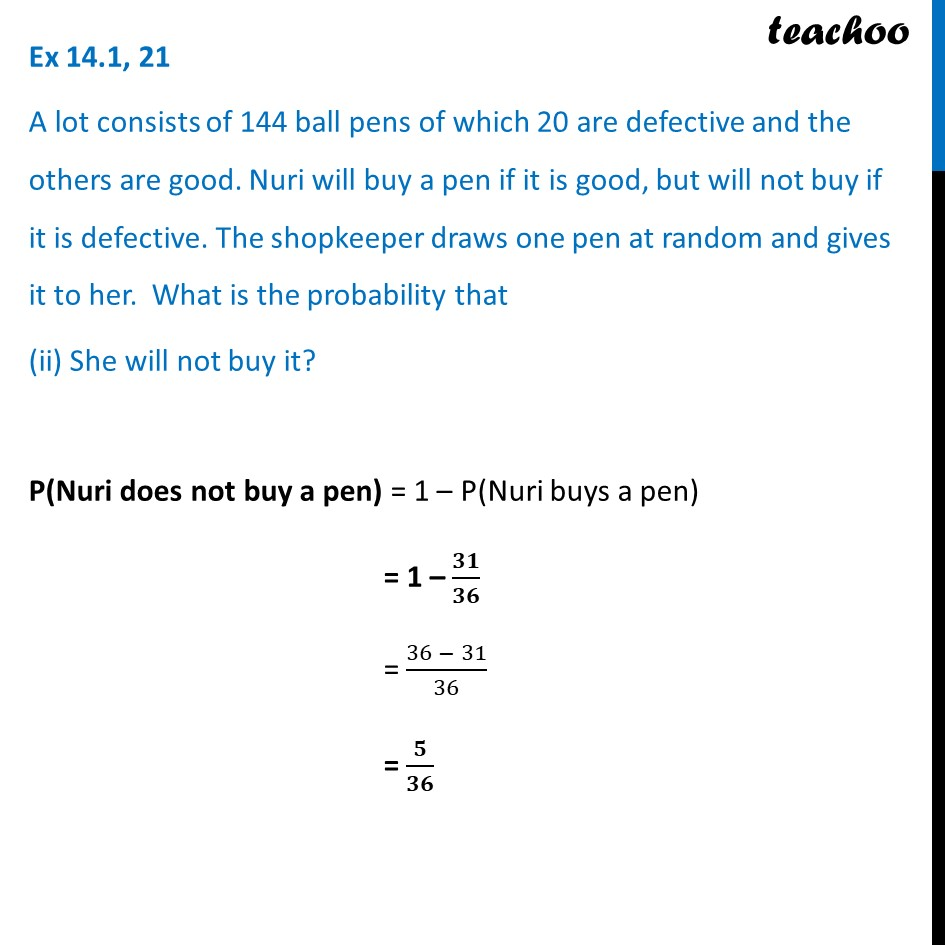Ex 15.1

Chapter 14 Class 10 Probability
Serial order wiseLearn in your speed, with individual attention - Teachoo Maths 1-on-1 Class

### Transcript

Ex 14.1, 21 A lot consists of 144 ball pens of which 20 are defective and the others are good. Nuri will buy a pen if it is good, but will not buy if it is defective. The shopkeeper draws one pen at random and gives it to her. What is the probability that She will buy it? Total number of pens = 144 Number of pens Nuri will buy = Number of good pens = Total number pens – Number of defective pens = 144 − 20 = 124 Now, P (Nuri buys a pen) = (𝑁𝑢𝑚𝑏𝑒𝑟 𝑜𝑓 𝑝𝑒𝑛𝑠 𝑁𝑢𝑟𝑖 𝑤𝑖𝑙𝑙 𝑏𝑢𝑦)/(𝑇𝑜𝑡𝑎𝑙 𝑁𝑢𝑚𝑏𝑒𝑟 𝑜𝑓 𝑝𝑒𝑛𝑠) = 124/144 = 𝟑𝟏/𝟑𝟔 Ex 14.1, 21 A lot consists of 144 ball pens of which 20 are defective and the others are good. Nuri will buy a pen if it is good, but will not buy if it is defective. The shopkeeper draws one pen at random and gives it to her. What is the probability that (ii) She will not buy it? P(Nuri does not buy a pen) = 1 – P(Nuri buys a pen) = 1 – 𝟑𝟏/𝟑𝟔 = (36 − 31)/36 = 𝟓/𝟑𝟔